Next: Investigation of Internal Up: Modeling of Physical Previous: Contrast Sensitivity of

Parameter Identification

Bert W. Rust, ACMD
Robert W. Ashton, Chemical Science and Technology Laboratory

An important inverse problem in time series modeling is to fit a system of ordinary differential equations (ODEs) to a set of observed time series data which are corrupted by stochastic measuring errors. Let the given data be one or more measured time series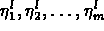, where the subscripts correspond to times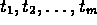and the superscript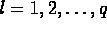is the time series index. Suppose that these data are to be modeled by a system of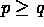first order ODEs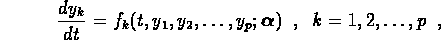where q of the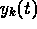are to be fit to the measured data, and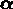is an n-vector of parameter values to be determined by fitting. If the initial measurements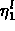are corrupted by measurement error, it is necessary to also include the p-vector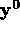of initial conditions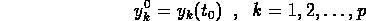as fitting parameters in order not to bias the fit. In most cases the system of ODEs cannot be solved in closed form so it is necessary to combine a numerical integrator with parameter fitting program. This is accomplished by using David Kahaner's integrator SDRIV1 together with the Stanford nonlinear least squares code VARPRO to minimize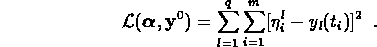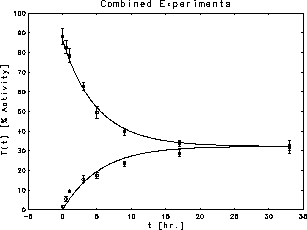Figure 13:  Simultaneous fits to the two thrombin concentration time series

The least squares code also requires the partial derivatives ofwith respect to each of the fitting parameters. These can be computed exactly by numerically integrating the system of variational equations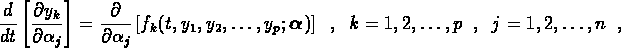and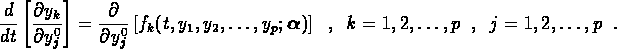Since each of theare implicit functions of all of the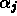and all of the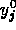, the partial derivatives of the right hand sides can become complicated and numerous.

A recent biochemical application of this program at NIST arose in connection with a study of the ability of anhydrothrombin (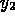), a derivative of the enzyme thrombin (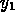), to compete with thrombin for the binding of a potent thrombin inhibitor hirudin (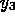). The system of ODEs describing the kinetics of the reactions can be written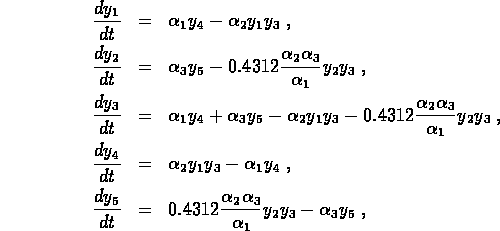where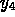and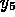are the concentrations of the molecules thrombin-hirudin complex and anhydrothrombin complex, respectively, and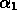,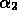and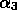are parameters to be determined by the fit. The initial values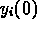were known exactly so there were only 15 variational equations for the partial derivatives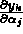.

The measured data were two time series of thrombin concentrations, corresponding to two separate experiments with different values of the initial conditions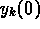. If the concentrations are expressed in units of %Activity, then one experiment started with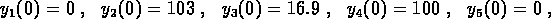and the other with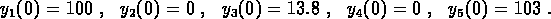The data for the two experiments were combined and the two time series were fit simultaneously to determine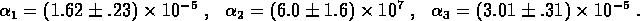The fits accounted for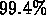of the combined total variance in the two measured records. The measurements and the fits are shown in Figure 13 where thrombin concentration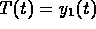is plotted against t measured in hours.Next: Investigation of Internal Up: Modeling of Physical Previous: Contrast Sensitivity of